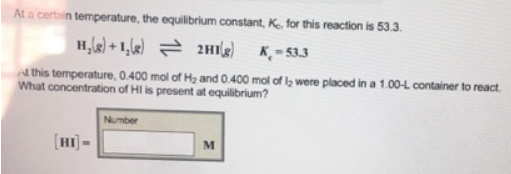# Problem: At a certain temperature, the equilibrium constant, Kc, for this reaction is 53.3. H2 (g) + I2 (g) ⇌ 2HI (g)             Kc = 53.3 At this temperature, 0.400 mol of H2 and 0.400 mol of I2 were placed in a 1.00 - L container to react. What concentration of HI is present at equilibrium?

###### FREE Expert Solution
90% (371 ratings)###### Problem Details

At a certain temperature, the equilibrium constant, Kc, for this reaction is 53.3.

H2 (g) + I2 (g) ⇌ 2HI (g)             Kc = 53.3

At this temperature, 0.400 mol of H2 and 0.400 mol of I2 were placed in a 1.00 - L container to react. What concentration of HI is present at equilibrium?